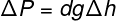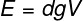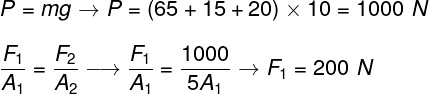Mechanics

# Hydrostatic

Hydrostatics is an area of ​​Physics that explains the behavior of fluids under static equilibrium conditions. This area involves applying concepts such as pressure and density through mathematical laws such as Pascal ‘s and Archimedes’ theorems . The knowledge derived from hydrostatics also allows us to better understand the functioning of hydraulic installations, as well as pipes, water tanks and even dams.

## Important concepts of hydrostatics

The most important concepts of hydrostatics are:

• density;
• pressure;
• buoyancy.

Together, they are sufficient to explain the way in which fluids at rest behave. To do so, we will only consider ideal fluids – non-compressible and only formed by molecules that do not interact with each other.

• ### hydrostatic pressure

Hydrostatic pressure is the pressure that a fluid exerts against the walls of its container. This pressure is directly proportional to the depth of that fluid – the deeper, the greater the pressure.

According to Pascal’s Theorem , pressure is evenly distributed throughout a fluid and all points that lie at the same depth are subject to the same pressure. One way to visualize this theorem in practice is to look at the shape acquired by a balloon or a ball. When full, they adopt a spherical shape, since inside the fluid (air, in this case), the pressure is distributed equally at all points.

The most commonly used units of pressure measurement are the pascal and the atm. The pascal is equivalent to a force of 1 newton applied to an area of ​​1 m², and the atm has the value of atmospheric pressure at sea level (about 1.10 5 Pa). Another unit, not as everyday as the atm, is the centimeter of mercury (cmHg) or even the millimeter of mercury (mmHg), which are still present in devices that measure blood pressure (sphygmomanometers) and also in cylinder pressure gauges. and gas cylinders.

• ### Density

Density is an important parameter for the study of fluids and for hydrostatics. It measures the amount of matter contained in a certain volume , that is, contained in the space occupied by a body or a fluid.

Density is defined based on pure water at room temperature. We attribute to this substance, which is abundant and easily found in any region of the planet, the density of 1 kg/L, 1 g/cm³ or 1000 kg/m³.

From the density of a substance, it is also possible to find out whether it will float or sink when dropped in water. Bodies denser than water sink, and bodies less dense than water float.

• ### Buoyancy

Buoyancy is the force that fluids exert on objects that are immersed in them . When we try to put a ball in water, we soon realize that a great force tends to push it out as it sinks.

The buoyancy is equal to the weight of the fluid that has been displaced due to the entry of a body into a fluid. This force points upwards and is dependent on the submerged volume ( the portion of the body that is inside the fluid), the density of the fluid, as well as the acceleration due to gravity .

## formulas

The main hydrostatic formulas are used to calculate parameters such as pressure and thrust. In the following figure, we present an important formula of hydrostatics, known as Stevin’s theorem . Watch:ΔP – pressure difference (atm, Pa)

d – fluid density (g/cm³, kg/m³)

Δh – height difference between two fluid points (cm, m)

The formula shown above can be used to determine the pressure difference between two points in a fluid that are at different heights. As we have seen earlier, points in fluids that are at the same height are subject to the same pressure. This behavior is described by Pascal’s theorem. Watch:P – pressure (Pa)

F – force (N)

A – area (m²)

From the formula above, we relate points 1 and 2 of a fluid located at the same depth by means of pressure, which can be calculated by the ratio of force to area .

Finally, we have the buoyancy formula, described by Archimedes’ theorem .E – thrust (N)

V – volume immersed, or volume of fluid displaced (m³)

## Summary

• Hydrostatics studies fluids at rest through variables such as pressure, volume and density.
• The pressure exerted by a fluid at rest is called hydrostatic pressure and is proportional to the depth of the fluid.
• Pressure is uniformly distributed within a fluid.
• When a body is immersed in a fluid, a force acts on it in order to expel it. This force is called buoyancy.
• Two points in a fluid at rest have the same pressure if they are at the same height.

## Solved exercises on hydrostatics

Question 1 — (Enem) To provide accessibility to people with limited mobility, the hydraulic elevator is used in buses and cars. In this device, an electric pump is used to force a fluid to pass from a narrow pipe to a wider one, and in this way activate a piston that moves the platform. Consider a hydraulic elevator whose piston head area is five times greater than the area of ​​the pipe leaving the pump. Neglecting friction and considering a gravitational acceleration of 10 m/s2, we want to lift a 65 kg person in a 15 kg wheelchair onto the 20 kg platform.

What force must be exerted by the pump motor on the fluid so that the wheelchair user is lifted at constant speed?

a) 20 N

b) 100 N

c) 200 N

d) 1000 N

e) 5000 N

Resolution:

To solve this exercise, we will make use of Pascal’s principle. Assuming that the pressure is evenly distributed throughout the fluid, the force-to-area ratio at both ends of the tube must be equal. Note the calculation:To make the above calculation, initially it was necessary to calculate the weight to be lifted by the hydraulic lift. After that, knowing that the second area is five times larger than the first, it was enough for us to make the division. Thus, the correct alternative is the letter C .

Question 2 — (Udesc) Consider the propositions related to hydrostatic fluids.

I. Pressure decreases with altitude above sea level and increases with depth below the air-water interface

II. The hydraulic lift is based on Pascal’s Principle

III. Knowing that the density of ice, oil and water are equal to 0.92 g/cm³, 0.80 g/cm³ and 1.0 g/cm³, respectively, it can be said that ice sinks in oil and floats on water

IV. The apparent weight of a completely immersed body is less than the real weight, due to the buoyant force exerted by the liquid on the body, from top to bottom.

Tick ​​the correct alternative.

a) Only statements I, II and III are true.

b) Only statements II and IV are true.

c) Only statements I and II are true.

d) Only statements I, III and IV are true.

e) All statements are true.

Resolution:

Analyzing the alternatives, it is clear that I, II and III are correct, based on the principles of hydrostatics, described in this article. The last alternative is incorrect because the buoyant force acts from the bottom up, not from the top down. Thus, the correct alternative is the letter A .